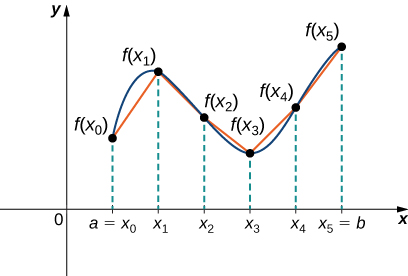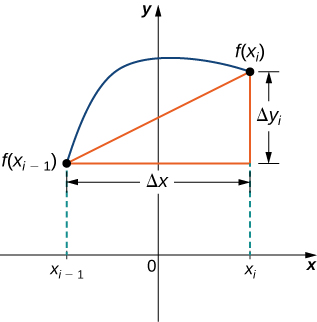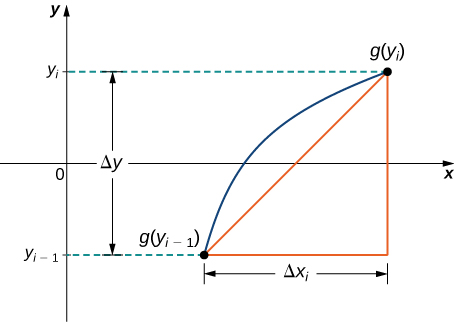## Arc Lengths of Curves

### Learning Outcomes

• Determine the length of a curve, $y=f(x),$ between two points
• Determine the length of a curve, $x=g(y),$ between two points

## Arc Length of the Curve $y$ = $f$($x$)

In previous applications of integration, we required the function $f(x)$ to be integrable, or at most continuous. However, for calculating arc length we have a more stringent requirement for $f(x).$ Here, we require $f(x)$ to be differentiable, and furthermore we require its derivative, ${f}^{\prime }(x),$ to be continuous. Functions like this, which have continuous derivatives, are called smooth. (This property comes up again in later chapters.)

Let $f(x)$ be a smooth function defined over $\left[a,b\right].$ We want to calculate the length of the curve from the point $(a,f(a))$ to the point $(b,f(b)).$ We start by using line segments to approximate the length of the curve. For $i=0,1,2\text{,…},n,$ let $P=\left\{{x}_{i}\right\}$ be a regular partition of $\left[a,b\right].$ Then, for $i=1,2\text{,…},n,$ construct a line segment from the point $({x}_{i-1},f({x}_{i-1}))$ to the point $({x}_{i},f({x}_{i})).$ Although it might seem logical to use either horizontal or vertical line segments, we want our line segments to approximate the curve as closely as possible. Figure 1 depicts this construct for $n=5.$Figure 1. We can approximate the length of a curve by adding line segments.

To help us find the length of each line segment, we look at the change in vertical distance as well as the change in horizontal distance over each interval. Because we have used a regular partition, the change in horizontal distance over each interval is given by $\text{Δ}x.$ The change in vertical distance varies from interval to interval, though, so we use $\text{Δ}{y}_{i}=f({x}_{i})-f({x}_{i-1})$ to represent the change in vertical distance over the interval $\left[{x}_{i-1},{x}_{i}\right],$ as shown in Figure 2. Note that some (or all) $\text{Δ}{y}_{i}$ may be negative.Figure 2. A representative line segment approximates the curve over the interval $\left[{x}_{i-1},{x}_{i}\right].$

By the Pythagorean theorem, the length of the line segment is $\sqrt{{(\text{Δ}x)}^{2}+{(\text{Δ}{y}_{i})}^{2}}.$ We can also write this as $\text{Δ}x\sqrt{1+{((\text{Δ}{y}_{i})\text{/}(\text{Δ}x))}^{2}}.$ Now, by the Mean Value Theorem, there is a point ${x}_{i}^{*}\in \left[{x}_{i-1},{x}_{i}\right]$ such that ${f}^{\prime }({x}_{i}^{*})=(\text{Δ}{y}_{i})\text{/}(\text{Δ}x).$ Then the length of the line segment is given by $\text{Δ}x\sqrt{1+{\left[{f}^{\prime }({x}_{i}^{*})\right]}^{2}}.$ Adding up the lengths of all the line segments, we get

$\text{Arc Length}\approx \underset{i=1}{\overset{n}{\text{∑}}}\sqrt{1+{\left[{f}^{\prime }({x}_{i}^{*})\right]}^{2}}\text{Δ}x.$

This is a Riemann sum. Taking the limit as $n\to \infty ,$ we have

$\text{Arc Length}=\underset{n\to \infty }{\text{lim}}\underset{i=1}{\overset{n}{\text{∑}}}\sqrt{1+{\left[{f}^{\prime }({x}_{i}^{*})\right]}^{2}}\text{Δ}x={\displaystyle\int }_{a}^{b}\sqrt{1+{\left[{f}^{\prime }(x)\right]}^{2}}dx.$

We summarize these findings in the following theorem.

### Arc Length for $y$ = $f$($x$)

Let $f(x)$ be a smooth function over the interval $\left[a,b\right].$ Then the arc length of the portion of the graph of $f(x)$ from the point $(a,f(a))$ to the point $(b,f(b))$ is given by

$\text{Arc Length}={\displaystyle\int }_{a}^{b}\sqrt{1+{\left[{f}^{\prime }(x)\right]}^{2}}dx.$

Note that we are integrating an expression involving ${f}^{\prime }(x),$ so we need to be sure ${f}^{\prime }(x)$ is integrable. This is why we require $f(x)$ to be smooth. The following example shows how to apply the theorem.

### Example: Calculating the Arc Length of a Function of $x$

Let $f(x)=2{x}^{3\text{/}2}.$ Calculate the arc length of the graph of $f(x)$ over the interval $\left[0,1\right].$ Round the answer to three decimal places.

### Try It

Let $f(x)=\left(\dfrac{4}{3}\right){x}^{3\text{/}2}.$ Calculate the arc length of the graph of $f(x)$ over the interval $\left[0,1\right].$ Round the answer to three decimal places.

Watch the following video to see the worked solution to the above Try It.

Although it is nice to have a formula for calculating arc length, this particular theorem can generate expressions that are difficult to integrate. We study some techniques for integration in Introduction to Techniques of Integration in the second volume of this text. In some cases, we may have to use a computer or calculator to approximate the value of the integral.

### Example: Using a Computer or Calculator to Determine the Arc Length of a Function of $x$

Let $f(x)={x}^{2}.$ Calculate the arc length of the graph of $f(x)$ over the interval $\left[1,3\right].$

### Try It

Let $f(x)= \sin x.$ Calculate the arc length of the graph of $f(x)$ over the interval $\left[0,\pi \right].$ Use a computer or calculator to approximate the value of the integral.

Watch the following video to see the worked solution to the above Try It.

## Arc Length of the Curve $x$ = $g$($y$)

We have just seen how to approximate the length of a curve with line segments. If we want to find the arc length of the graph of a function of $y,$ we can repeat the same process, except we partition the $y\text{-axis}$ instead of the $x\text{-axis}.$ Figure 3 shows a representative line segment.Figure 3. A representative line segment over the interval $\left[{y}_{i-1},{y}_{i}\right].$

Then the length of the line segment is $\sqrt{{(\text{Δ}y)}^{2}+{(\text{Δ}{x}_{i})}^{2}},$ which can also be written as $\text{Δ}y\sqrt{1+{((\text{Δ}{x}_{i})\text{/}(\text{Δ}y))}^{2}}.$ If we now follow the same development we did earlier, we get a formula for arc length of a function $x=g(y).$

### Arc Length for $x$ = $g$($y$)

Let $g(y)$ be a smooth function over an interval $\left[c,d\right].$ Then, the arc length of the graph of $g(y)$ from the point $(c,g(c))$ to the point $(d,g(d))$ is given by

$\text{Arc Length}={\displaystyle\int }_{c}^{d}\sqrt{1+{\left[{g}^{\prime }(y)\right]}^{2}}dy$

### Example: Calculating the Arc Length of a Function of $y$

Let $g(y)=3{y}^{3}.$ Calculate the arc length of the graph of $g(y)$ over the interval $\left[1,2\right].$

### Try It

Let $g(y)=\frac{1}{y}.$ Calculate the arc length of the graph of $g(y)$ over the interval $\left[1,4\right].$ Use a computer or calculator to approximate the value of the integral.